### Home > MC1 > Chapter 3 > Lesson 3.2.3 > Problem3-53

3-53.

Copy and complete each of the Diamond Problems below.
The pattern used in the Diamond Problems is shown at right.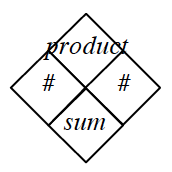1.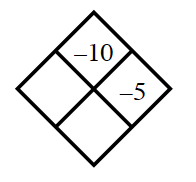Find the correct number for the left side of the diamond first. You'll know you are correct when the product equals $-10$.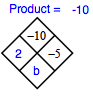Don't forget to find the sum!

1.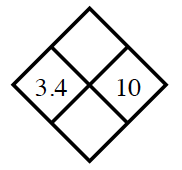Using your addition and multiplication skills, try finding the sum and product of the numbers above.

See the diamond for the correct answers.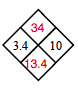1.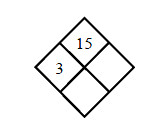Using division, first try to find the value of c, the number in the right of the diamond.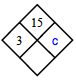1.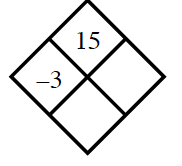Follow the pattern to find the value of d. Once you have d, add it to $-3$ to find e.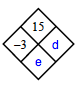The value of d is $−5$ and the sum (e) is $−8$.